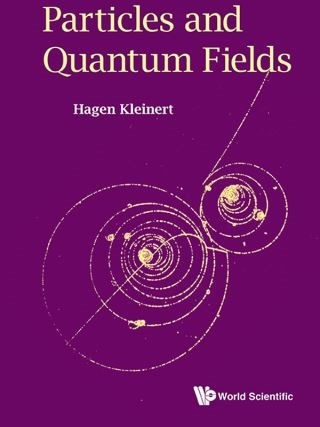## Particles and Quantum Fields• Description
• Author
• Info
• Reviews

### Description

This is an introductory book on elementary particles and their interactions. It starts out with many-body Schrödinger theory and second quantization and leads, via its generalization, to relativistic fields of various spins and to gravity. The text begins with the best known quantum field theory so far, the quantum electrodynamics of photon and electrons (QED). It continues by developing the theory of strong interactions between the elementary constituents of matter (quarks). This is possible due to the property called asymptotic freedom. On the way one has to tackle the problem of removing various infinities by renormalization. The divergent sums of infinitely many diagrams are performed with the renormalization group or by variational perturbation theory (VPT). The latter is an outcome of the Feynman-Kleinert variational approach to path integrals discussed in two earlier books of the author, one representing a comprehensive treatise on path integrals, the other dealing with critial phenomena. Unlike ordinary perturbation theory, VPT produces uniformly convergent series which are valid from weak to strong couplings, where they describe critical phenomena.

The present book develops the theory of effective actions which allow to treat quantum phenomena with classical formalism. For example, it derives the observed anomalous power laws of strongly interacting theories from an extremum of the action. Their fluctuations are not based on Gaussian distributions, as in the perturbative treatment of quantum field theories, or in asymptotically-free theories, but on deviations from the average which are much larger and which obey power-like distributions.

Exactly solvable models are discussed and their physical properties are compared with those derived from general methods. In the last chapter we discuss the problem of quantizing the classical theory of gravity.

Contents:
• Fundamentals
• Field Formulation of Many-Body Quantum Physics
• Interacting Nonrelativistic Particles
• Free Relativistic Particles and Fields
• Relativistic Particles and Fields in External Electromagnetic Potential
• Quantization of Relativistic Free Fields
• Continuous Symmetries and Conservation Laws. Noether's Theorem
• Scattering and Decay of Particles
• Quantum Field Theoretic Perturbation Theory
• Extracting Finite Results from Perturbation Series. Regularization, Renormalization
• Quantum Electrodynamics
• Formal Properties of Perturbation Theory
• Functional-Integral Representation of Quantum Field Theory
• Systematic Graphical Construction of Feynman Diagrams
• Spontaneous Symmetry Breakdown
• Scalar Quantum Electrodynamics
• Exactly Solvable O(N)-Symmetric ϕ4-Theory for Large N
• Nonlinear σ-Model
• The Renormalization Group
• Critical Properties of Nonlinear σ-Model
• Functional-Integral Calculation of Effective Action. Loop Expansion
• Exactly Solvable O(N)-Symmetric Four-Fermion Theory in 2+ε Dimensions
• Internal Symmetries of Strong Interactions
• Symmetries Linking Internal and Spacetime Properties
• Weak Interactions
• Nonabelian Gauge Theory of Strong Interactions
• Cosmology with General Curvature-Dependent Lagrangian
• Einstein Gravity from Fluctuating Conformal Gravity
• Purely Geometric Part of Dark Matter

Readership: Students and researchers in theoretical physics.